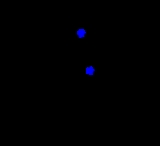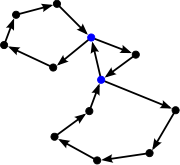xPath (graph theory)Encyclopedia
In graph theory
Graph theory
In mathematics and computer science, graph theory is the study of graphs, mathematical structures used to model pairwise relations between objects from a certain collection. A "graph" in this context refers to a collection of vertices or 'nodes' and a collection of edges that connect pairs of...

, a path in a graph
Graph (mathematics)
In mathematics, a graph is an abstract representation of a set of objects where some pairs of the objects are connected by links. The interconnected objects are represented by mathematical abstractions called vertices, and the links that connect some pairs of vertices are called edges...

is a sequence
Sequence
In mathematics, a sequence is an ordered list of objects . Like a set, it contains members , and the number of terms is called the length of the sequence. Unlike a set, order matters, and exactly the same elements can appear multiple times at different positions in the sequence...

of vertices
Vertex (graph theory)
In graph theory, a vertex or node is the fundamental unit out of which graphs are formed: an undirected graph consists of a set of vertices and a set of edges , while a directed graph consists of a set of vertices and a set of arcs...

such that from each of its vertices there is an edge to the next vertex in the sequence. A path may be infinite, but a finite path always has a first vertex, called its start vertex, and a last vertex, called its end vertex. Both of them are called terminal vertices of the path. The other vertices in the path are internal vertices. A cycle
Cycle (graph theory)
In graph theory, the term cycle may refer to a closed path. If repeated vertices are allowed, it is more often called a closed walk. If the path is a simple path, with no repeated vertices or edges other than the starting and ending vertices, it may also be called a simple cycle, circuit, circle,...

is a path such that the start vertex and end vertex are the same. The choice of the start vertex in a cycle is arbitrary.Paths and cycles are fundamental concepts of graph theory, described in the introductory sections of most graph theory texts. See e.g. Bondy and Murty (1976), Gibbons (1985), or Diestel (2005). Korte et al. (1990) cover more advanced algorithmic topics concerning paths in graphs.

The vertices of a path are said to be connected. The vertices of a directed cycle are said to be strongly connected.

## Different types of path

The same concepts apply both to undirected graphs and directed graph
Directed graph
A directed graph or digraph is a pair G= of:* a set V, whose elements are called vertices or nodes,...

s, with the edges being directed from each vertex to the following one. Often the terms directed path and directed cycle are used in the directed case.

A path with no repeated vertices is called a simple path, and a cycle with no repeated vertices or edges aside from the necessary repetition of the start and end vertex is a simple cycle. In modern graph theory
Graph theory
In mathematics and computer science, graph theory is the study of graphs, mathematical structures used to model pairwise relations between objects from a certain collection. A "graph" in this context refers to a collection of vertices or 'nodes' and a collection of edges that connect pairs of...

, most often "simple" is implied; i.e., "cycle" means "simple cycle" and "path" means "simple path", but this convention is not always observed, especially in applied graph theory. Some authors (e.g. Bondy and Murty 1976) use the term "walk" for a path in which vertices or edges may be repeated, and reserve the term "path" for what is here called a simple path.

A path such that no graph edges connect two nonconsecutive path vertices is called an induced path
Induced path
In the mathematical area of graph theory, an induced path in an undirected graph G is a path that is an induced subgraph of G. That is, it is a sequence of vertices in G such that each two adjacent vertices in the sequence are connected by an edge in G, and each two nonadjacent vertices in the...

.

A simple cycle that includes every vertex, without repetition, of the graph is known as a Hamiltonian cycle.

A cycle with just one edge removed in the corresponding spanning tree
Spanning tree
Spanning tree can refer to:* Spanning tree , a tree which contains every vertex of a more general graph* Spanning tree protocol, a protocol for finding spanning trees in bridged networks...

of the original graph is known as a Fundamental cycle.
Two paths are vertex-independent (alternatively, internally vertex-disjoint) if they do not have any internal vertex in common. Similarly, two paths are edge-independent (or edge-disjoint) if they do not have any internal edge in common.

The length of a path is the number of edges that the path uses, counting multiple edges multiple times. The length can be zero for the case of a single vertex.

A weighted graph associates a value (weight) with every edge in the graph. The weight of a path in a weighted graph is the sum of the weights of the traversed edges. Sometimes the words cost or length are used instead of weight.

• Glossary of graph theory
Glossary of graph theory
Graph theory is a growing area in mathematical research, and has a large specialized vocabulary. Some authors use the same word with different meanings. Some authors use different words to mean the same thing. This page attempts to keep up with current usage....

• Shortest path problem
Shortest path problem
In graph theory, the shortest path problem is the problem of finding a path between two vertices in a graph such that the sum of the weights of its constituent edges is minimized...

• Traveling salesman problem
• Cycle space
Cycle space
In graph theory, an area of mathematics, a cycle space is a vector space defined from an undirected graph; elements of the cycle space represent formal combinations of cycles in the graph....